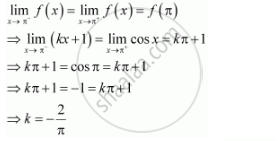Share

# Find the Values Of K So that the Function F Is Continuous at the Indicated Point. F(X) = {(Kx +1, If X<= Pi),(Cos X, If X > Pi) At X = Pi - CBSE (Commerce) Class 12 - Mathematics

#### Question

Find the values of so that the function f is continuous at the indicated point.

f(x) = {(kx +1, if x<= pi),(cos x, if x > pi):} " at  x " = pi

#### Solution

The given function is f(x) = {(kx +1, "," if x<= pi),(cos x, ", "if x > pi):}

The given function f is continuous at x = p, if f is defined at x = p and if the value of fat x = p equals the limit of f at x = p

It is evident that is defined at x = p and f(pi) = kpi + 1Therefore, the required value of k  is -2/pi

Is there an error in this question or solution?

#### APPEARS IN

NCERT Solution for Mathematics Textbook for Class 12 (2018 to Current)
Chapter 5: Continuity and Differentiability
Q: 28 | Page no. 161

#### Video TutorialsVIEW ALL 

Solution Find the Values Of K So that the Function F Is Continuous at the Indicated Point. F(X) = {(Kx +1, If X<= Pi),(Cos X, If X > Pi) At X = Pi Concept: Algebra of Continuous Functions.
S